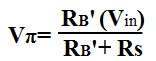﻿ How to Calculate Vπ (Vpi) of a Transistor

# How to Calculate Vπ (Vpi) of a Transistor?

Vπ, the voltage that drops across the resistor, rπ, of a transistor, is an important calculation to make because it is the voltage in parallel with the current amplifier and the output resistors. The amplified current signal of a transistor is usually calculated by the formula I=gmVπ, so it is important to know how to find the value of Vπ.

Vπ is calculated by doing AC analysis of the transistor circuit. The AC model of the circuit needs to be created. From there, voltage, Vπ, can be derived.

Below is the AC equivalent of transistor circuit:We calculate Vπ by narrowing down the left side of the circuit:

We first solve for R1||R2, which is RB. The formula is below:Next we solve for RB||rπ, which is RB'. The formula is below:After this, our circuit gets reduced to the circuit below:Now doing a simple KVL loop around the left hand of the transistor circuit, we calculate Vπ to be: